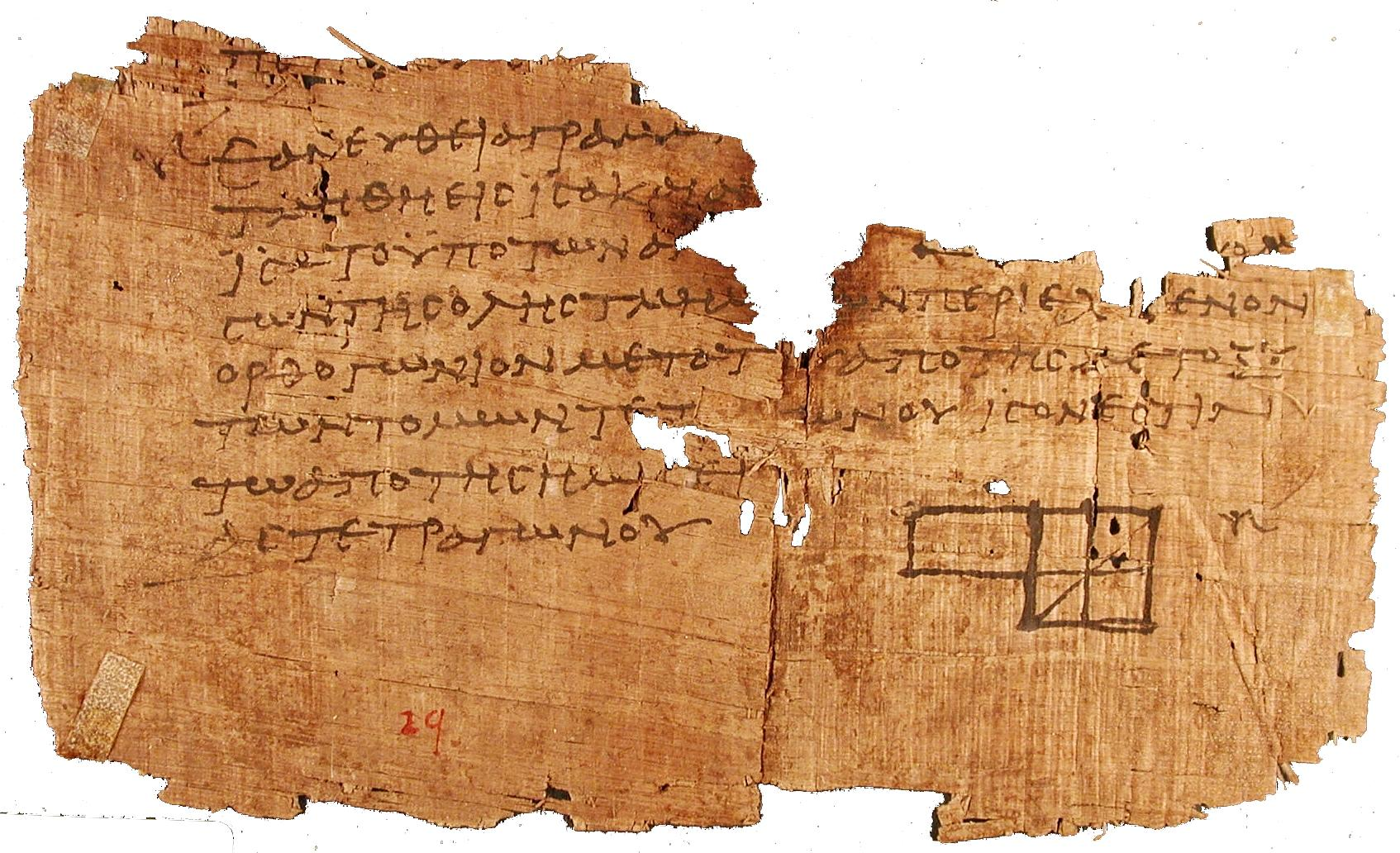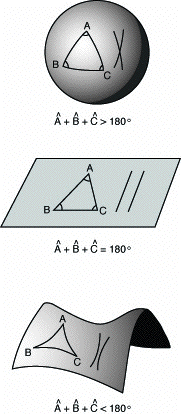# Tag Archives: Euclid# Non-Euclidean Geometries

This post is an adaptation of a chapter of my book  “The Wraparound Universe” with many more  illustrations.

************************************************************************

Thus we may perhaps, one day, create new Figures
that will allow us to put our trust in the Word,
in order to traverse curved Space, non-Euclidean Space.
Francis PongeThe oldest known fragment of Euclid’s Elements as part of the Oxyrhynchus papyri, dated from the Ptolemaic period and belonging to the famous Alexandrian Library

In book I of the Elements, Euclid poses the five “requests” that, according to him, define planar geometry. These postulates would become the keystone for all of geometry, a system of absolute truths whose validity seemed irrefutable. One of the reasons for this faith is that these postulates seem obvious: the first of them stipulates that a straight line passes between two points, the second that any line segment can be indefinitely prolonged in both directions, the third that, given a point and an interval, it is always possible to trace out a circle having the point for its center and the interval as its radius, the fourth that all right angles are equal to each other. The fifth postulate is however less obvious:As the sum of the interior angles α and β is less than 180°, according to the fifth postulate the two straight lines extended indefinitely, meet on that side.

“If a line segment intersects two straight lines forming two interior angles on the same side that sum to less than two right angles, then the two lines, if extended indefinitely, meet on that side on which the angles sum to less than two right angles.”

Although the statement does not refer explicitly to parallel lines, the the fifth postulate is currently called “Parallel postulate”. This can be better understood given the more popular version of the fifth postulate due to the Scottish mathematician John Playfair (1748-1819), who demonstrated that it was equivalent to the one given by Euclid : “Given a straight line and a point not belonging to this line, there exists a unique straight line passing through the point which is parallel to the first“.Since the “parallel postulate” was more complicated than the others, the mathematicians following Euclid would try, for many centuries, to prove it from the four preceding ones, all in vain. In the nineteenth century, there occurred one of the great sudden revolutions in the history of mathematics (and also in human thought, as will be seen by what follows): two new geometries which do not satisfy the fifth postulate, but which are perfectly coherent, were discovered. In one of these geometries, called spherical geometry, no parallel line satisfying the conditions can be traced. This is the case for the surface of a sphere; the straight lines become great circles, whose planes pass through the center of the sphere, and since all great circles intersect each other at two diametrically opposed points (in the manner of the terrestrial meridians, which meet at the poles), no “straight line” can be parallel to another. In the other geometry, called hyperbolic geometry, through any given point there passes an infinite number of lines parallel to another straight line. Continue reading